# 算法路漫漫(一) 简单排序

## 1.认识时间复杂度

`常数时间的操作：一个操作如果和数据量没有关系，每次都是固定时间内完成的操作，叫做常数操作。时间复杂度为一个算法流程中，常数操作数量的指标。常用O（读作big O）来表示。具体来说，在常数操作数量的表达式中，只要高阶项，不要低阶项，也不要高阶项的系数，剩下的部分如果记为f(N)，那么时间复杂度为O(f(N))。评价一个算法流程的好坏，先看时间复杂度的指标，然后再分析不同数据样本下的实际运行时间，也就是常数项时间。`

### 衡量算法复杂度

```1.内存（Memory）
2.时间（Time）
3.指令的数量（Number of Steps）
4.特定操作的数量
磁盘访问数量
网络包数量
5.渐进复杂度（Asymptotic Complexity）```

### 算法的运行时间与什么相关

```1.输入的数据。（例如：如果数据已经是排好序的，时间消耗可能会减少。）
2.输入数据的规模。（例如：6 和 6 * 109）
3.运行时间的上限。（因为运行时间的上限是对使用者的承诺。）```

```1.忽略掉那些依赖于机器的常量。
2.关注运行时间的增长趋势。```

```1.线性复杂度 O(n) 表示每个元素都要被处理一次。
2.平方复杂度 O(n2) 表示每个元素都要被处理 n 次。```
 Notation Intuition Informal Definition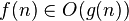f is bounded above by g asymptotically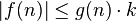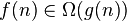Two definitions :Number theory: f is not dominated by g asymptotically Complexity theory: f is bounded below by g asymptotically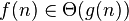f is bounded both above and below by g asymptotically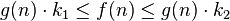## 2.简单排序

### 2.1 选择排序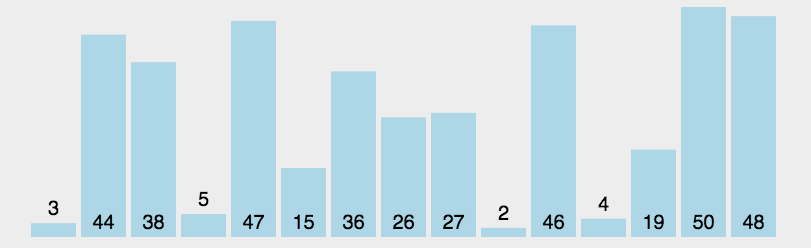```    private void sellectionSorted(int[] arr){
if(arr == null || arr.length <=1){
return;
}
// i ~ N-1
for(int i=0;i<arr.length-1;i++){
int minIndex=i;
// i ~ N-1 get min
for(int j=i+1;j<arr.length;j++){
minIndex = arr[j] < arr[minIndex] ? j : minIndex;
}
swap(arr ,minIndex,i);
}
}

private void swap(int[] arr, int a, int b){
int tmp = arr[a];
arr[a] = arr[b];
arr[b] = tmp;
}```

### 2.2 冒泡排序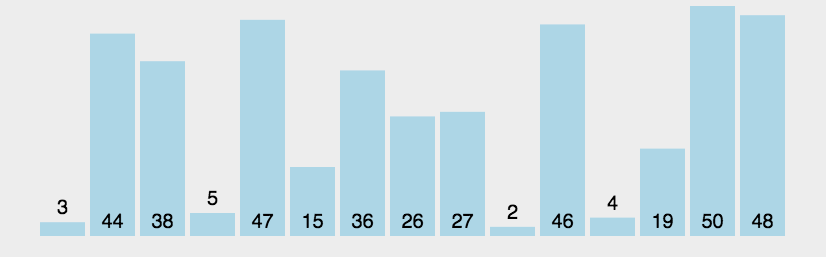```    private void bubbleSorted(int[] arr){
if(arr == null || arr.length <=1){
return;
}
// i ~ N-1
for(int i=arr.length-1;i>0;i--){
// i ~ N-1 set max to the end of arr
for(int j=0;j<i;j++){
if(arr[j+1] < arr[j]) {
swap(arr ,j+1,j);
}
}

}
}

// 异或运算
private void swap(int[] arr, int a, int b){
arr[a] = arr[a]^arr[b];
arr[b] = arr[a]^arr[b];
arr[a] = arr[a]^arr[b];
}```

### 2.3 插入排序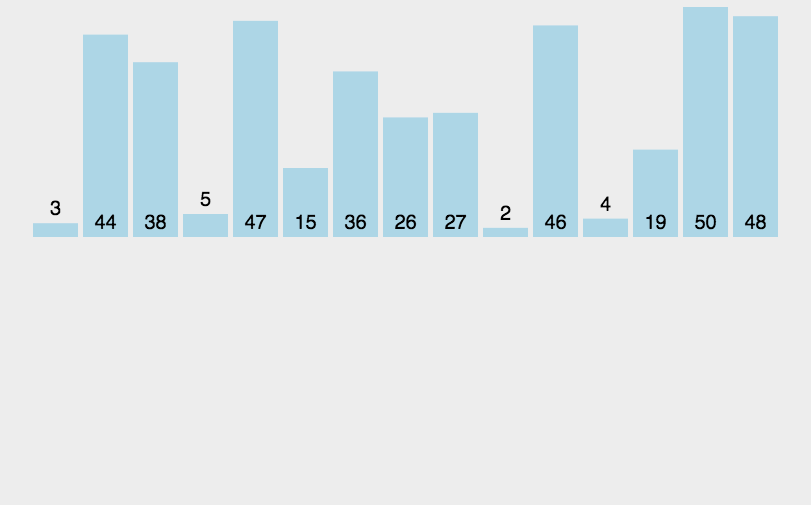```    private void insertSorted(int[] arr){
if(arr == null || arr.length <=1){
return;
}
// 0 ~1 compare and swap
// 0 ~2 compare and swap
for(int i=0;i<arr.length;i++){
// i ~ N-1 compare and swap
for(int j=i;j>0;j--){
if(arr[j-1] > arr[j]) {
swap(arr ,j-1,j);
}
}

}
}

// 异或运算
private void swap(int[] arr, int a, int b){
arr[a] = arr[a]^arr[b];
arr[b] = arr[a]^arr[b];
arr[a] = arr[a]^arr[b];
}```

### 2.4 二分法

```    private int searchMatch(int[] arr, int low, int high, int target){
if(low > high){
return -1;
}
int mid = low + ((high-low)>>1);

//
if(arr[mid] == target){
return mid;
} else if(arr[mid] < target){
// search in [mid+1, high]
return searchMatch(arr, mid+1, high, target);
} else {
// search in [low, mid-1]
return searchMatch(arr, low, mid-1, target);
}
}```

```1.在一个有序数组中，查找某个数是否存在

2.在一个有序数组中，查找>=某个数最左侧位置

3. 在一个无序数组中，任何相邻两数不相等，寻找局部最小值```

## 3.补充

### 3.1 异或运算

```1. 0^N=N, N^N=02.异或运算满足交换率和结合率A^B = B^A, A^(B^C) = (A^B)^C
3.同样一批数，异或运算，先后顺序变化 不影响结果A^B^...^Z = Z^Y^...^A
4.无进位加法，相同为0，不同为1；int a= 3= 011int b= 5= 101int c= a^b = 011^101=110=6```

### 3.2 取出一个数对应二进制最右侧的1对应的整数

`// 与上自己的取反加1int a= A & (～A+1)；`

### 3.3 对数器

```1.有一个你想要测的方法A
2.实现一个绝对正确但是复杂度不好的方法B
3.实现一个随机样本产生器
4.实现比对的方法
5.把方法A和方法B比对很多次来验证方法B是否正确。
6.如果有一个样本使得比对出错，打印样本分析是哪个方法出错
7.当样本数量很多时比对测试(假设10万次测试)依然正确，可以确定方法A已经正确```

(0)
(0)